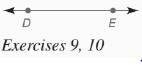Chapter 7.CR, Problem 9CR### Elementary Geometry for College St...

6th Edition
Daniel C. Alexander + 1 other
ISBN: 9781285195698

#### Solutions

Chapter
Section### Elementary Geometry for College St...

6th Edition
Daniel C. Alexander + 1 other
ISBN: 9781285195698
Textbook Problem
1 views

# In Review Exercises 7 to 13, sketch and describe the locus in a plane.Find the locus of points equidistant from points D and E .To determine

To find: The locus of points that are equidistant from points D and E.

Explanation

Given:

The line with points D and E is shown below,

Definition used:

A locus is the set of all points and only those points that satisfy a given condition (or set of conditions).

Calculation:

Consider the given figure.

The locus is the line that is the perpendicular bisector of DE¯<

### Still sussing out bartleby?

Check out a sample textbook solution.

See a sample solution

#### The Solution to Your Study Problems

Bartleby provides explanations to thousands of textbook problems written by our experts, many with advanced degrees!

Get Started